How Cheenta works to ensure student success?
Explore the Back-Story

# Test of Mathematics Solution Subjective 83 - Two numbers adding up to 1This is a Test of Mathematics Solution Subjective 83 (from ISI Entrance). The book, Test of Mathematics at 10+2 Level is Published by East West Press. This problem book is indispensable for the preparation of I.S.I. B.Stat and B.Math Entrance.

## Problem

If a and b are positive real numbers such that a + b = 1, prove that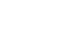+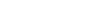## Solution:

We replace 1 by a+b and expand the squares.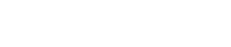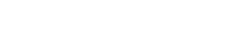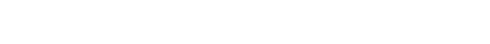Now we use A.M. - G.M. inequality to have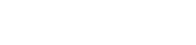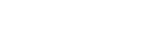Also we apply the square mean inequality to have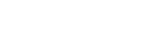Since a+b = 1, we have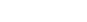Combining all of them we have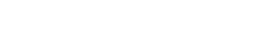Discussion

Some theoretical background.This is a Test of Mathematics Solution Subjective 83 (from ISI Entrance). The book, Test of Mathematics at 10+2 Level is Published by East West Press. This problem book is indispensable for the preparation of I.S.I. B.Stat and B.Math Entrance.

## Problem

If a and b are positive real numbers such that a + b = 1, prove that+## Solution:

We replace 1 by a+b and expand the squares.Now we use A.M. - G.M. inequality to haveAlso we apply the square mean inequality to haveSince a+b = 1, we haveCombining all of them we haveDiscussion

Some theoretical background.

### Knowledge Partner